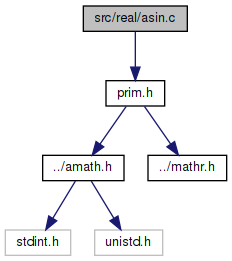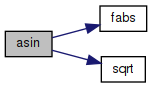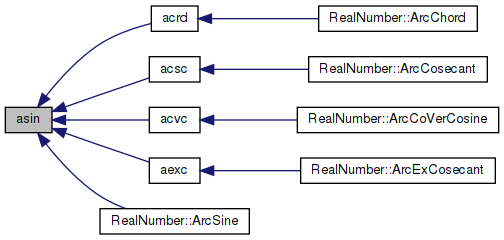amath  1.8.5 Simple command line calculator
asin.c File Reference

Inverse sine function. More...

`#include "prim.h"`
Include dependency graph for asin.c:Go to the source code of this file.

## Functions

double asin (double x)
Inverse sine function. More...

## Variables

static const double one = 1.00000000000000000000e+00

static const double huge = 1.000e+300

static const double pio2_hi = 1.57079632679489655800e+00

static const double pio2_lo = 6.12323399573676603587e-17

static const double pio4_hi = 7.85398163397448278999e-01

static const double pS0 = 1.66666666666666657415e-01

static const double pS1 = -3.25565818622400915405e-01

static const double pS2 = 2.01212532134862925881e-01

static const double pS3 = -4.00555345006794114027e-02

static const double pS4 = 7.91534994289814532176e-04

static const double pS5 = 3.47933107596021167570e-05

static const double qS1 = -2.40339491173441421878e+00

static const double qS2 = 2.02094576023350569471e+00

static const double qS3 = -6.88283971605453293030e-01

static const double qS4 = 7.70381505559019352791e-02

## Detailed Description

Inverse sine function.

Definition in file asin.c.

## ◆ asin()

 double asin ( double x )

Inverse sine function.

`Method`
```Since  asin(x) = x + x^3/6 + x^5*3/40 + x^7*15/336 + ...
we approximate asin(x) on [0,0.5] by
asin(x) = x + x*x^2*R(x^2)
where
R(x^2) is a rational approximation of (asin(x)-x)/x^3
and its remez error is bounded by
|(asin(x)-x)/x^3 - R(x^2)| < 2^(-58.75)```
```For x in [0.5,1]
asin(x) = pi/2-2*asin(sqrt((1-x)/2))
Let y = (1-x), z = y/2, s := sqrt(z), and pio2_hi+pio2_lo=pi/2;
then for x>0.98
asin(x) = pi/2 - 2*(s+s*z*R(z))
= pio2_hi - (2*(s+s*z*R(z)) - pio2_lo)```
```For x<=0.98, let pio4_hi = pio2_hi/2, then
f = hi part of s;
c = sqrt(z) - f = (z-f*f)/(s+f)     ...f+c=sqrt(z)
and
asin(x) = pi/2 - 2*(s+s*z*R(z))
= pio4_hi+(pio4-2s)-(2s*z*R(z)-pio2_lo)
= pio4_hi+(pio4-2f)-(2s*z*R(z)-(pio2_lo+2c))```
```Special cases
if x is NaN, return NaN
if |x|>1, return NaN
```

Definition at line 100 of file asin.c.

References fabs(), huge, one, pio2_hi, pio2_lo, pio4_hi, pS0, pS1, pS2, pS3, pS4, pS5, qS1, qS2, qS3, qS4, and sqrt().

Referenced by acrd(), acsc(), acvc(), aexc(), and RealNumber::ArcSine().

101 {
102  double t, w, p, q, c, r, s;
103  int32_t hx, ix;
104  GET_HIGH_WORD(hx, x);
105  ix = hx & 0x7fffffff;
106  if (ix >= 0x3ff00000)
107  { /* |x|>= 1 */
108  uint32_t lx;
109  GET_LOW_WORD(lx, x);
110  if (((ix - 0x3ff00000) | lx) == 0)
111  /* asin(1)=+-pi/2 with inexact */
112  return x * pio2_hi + x * pio2_lo;
113  return NAN; /* asin(|x|>1) is NaN */
114  }
115  else if (ix < 0x3fe00000)
116  { /* |x|<0.5 */
117  if (ix < 0x3e400000)
118  { /* if |x| < 2**-27 */
119  if (huge + x > one)
120  {
121  return x; /* return x with inexact if x!=0*/
122  }
123  else
124  {
125  t = 0;
126  }
127  }
128  else
129  {
130  t = x * x;
131  }
132
133  p = t * (pS0 + t * (pS1 + t * (pS2 + t * (pS3 + t * (pS4 + t * pS5)))));
134  q = one + t * (qS1 + t * (qS2 + t * (qS3 + t * qS4)));
135  w = p / q;
136  return x + x * w;
137  }
138  /* 1> |x|>= 0.5 */
139  w = one - fabs(x);
140  t = w * 0.5;
141  p = t * (pS0 + t * (pS1 + t * (pS2 + t * (pS3 + t * (pS4 + t * pS5)))));
142  q = one + t * (qS1 + t * (qS2 + t * (qS3 + t * qS4)));
143  s = sqrt(t);
144  if (ix >= 0x3FEF3333)
145  { /* if |x| > 0.975 */
146  w = p / q;
147  t = pio2_hi - (2.0 * (s + s * w) - pio2_lo);
148  }
149  else
150  {
151  w = s;
152  SET_LOW_WORD(w, 0);
153  c = (t - w * w) / (s + w);
154  r = p / q;
155  p = 2.0 * s * r - (pio2_lo - 2.0 * c);
156  q = pio4_hi - 2.0 * w;
157  t = pio4_hi - (p - q);
158  }
159  if (hx > 0)
160  return t;
161  else
162  return -t;
163 }
static const double pS1
Definition: asin.c:56
#define GET_HIGH_WORD(i, d)
Get the more significant 32 bit int from a double.
Definition: prim.h:167
static const double huge
Definition: asin.c:50
static const double pS4
Definition: asin.c:59
static const double pS5
Definition: asin.c:60
static const double pio2_hi
Definition: asin.c:51
#define GET_LOW_WORD(i, d)
Get the less significant 32 bit int from a double.
Definition: prim.h:177
static const double qS1
Definition: asin.c:61
static const double pio4_hi
Definition: asin.c:53
static const double pS0
Definition: asin.c:55
#define NAN
Definition: mathr.h:53
static const double pS2
Definition: asin.c:57
static const double qS4
Definition: asin.c:64
static const double one
Definition: asin.c:49
static const double pS3
Definition: asin.c:58
static const double pio2_lo
Definition: asin.c:52
#define SET_LOW_WORD(d, v)
Set the less significant 32 bits of a double from an int.
Definition: prim.h:209
double sqrt(double x)
Square root function.
Definition: sqrt.c:119
static const double qS2
Definition: asin.c:62
double fabs(double x)
Returns the absolute value of x.
Definition: fabs.c:51
static const double qS3
Definition: asin.c:63
Here is the call graph for this function:Here is the caller graph for this function:## ◆ huge

 const double huge = 1.000e+300
static

Definition at line 50 of file asin.c.

Referenced by asin().

## ◆ one

 const double one = 1.00000000000000000000e+00
static

Definition at line 49 of file asin.c.

Referenced by asin().

## ◆ pio2_hi

 const double pio2_hi = 1.57079632679489655800e+00
static

Definition at line 51 of file asin.c.

Referenced by asin().

## ◆ pio2_lo

 const double pio2_lo = 6.12323399573676603587e-17
static

Definition at line 52 of file asin.c.

Referenced by asin().

## ◆ pio4_hi

 const double pio4_hi = 7.85398163397448278999e-01
static

Definition at line 53 of file asin.c.

Referenced by asin().

## ◆ pS0

 const double pS0 = 1.66666666666666657415e-01
static

Definition at line 55 of file asin.c.

Referenced by asin().

## ◆ pS1

 const double pS1 = -3.25565818622400915405e-01
static

Definition at line 56 of file asin.c.

Referenced by asin().

## ◆ pS2

 const double pS2 = 2.01212532134862925881e-01
static

Definition at line 57 of file asin.c.

Referenced by asin().

## ◆ pS3

 const double pS3 = -4.00555345006794114027e-02
static

Definition at line 58 of file asin.c.

Referenced by asin().

## ◆ pS4

 const double pS4 = 7.91534994289814532176e-04
static

Definition at line 59 of file asin.c.

Referenced by asin().

## ◆ pS5

 const double pS5 = 3.47933107596021167570e-05
static

Definition at line 60 of file asin.c.

Referenced by asin().

## ◆ qS1

 const double qS1 = -2.40339491173441421878e+00
static

Definition at line 61 of file asin.c.

Referenced by asin().

## ◆ qS2

 const double qS2 = 2.02094576023350569471e+00
static

Definition at line 62 of file asin.c.

Referenced by asin().

## ◆ qS3

 const double qS3 = -6.88283971605453293030e-01
static

Definition at line 63 of file asin.c.

Referenced by asin().

## ◆ qS4

 const double qS4 = 7.70381505559019352791e-02
static

Definition at line 64 of file asin.c.

Referenced by asin().